# Solutions for Class 7 Mathematics

• ### NCERT Solutions Class 7 Mathematics Visualizing Solid Shapes

NCERT Solutions Class 7 Mathematics Visualizing Solid Shapes. The NCERT solutions have been made by teacher of one of the best CBSE school in India. These solutions have been made to give detailed answers and expanations of the concepts which can be easily understood by the students. Refer to...

• ### NCERT Solutions Class 7 Mathematics Triangle and its properties

NCERT Solutions Class 7 Mathematics Triangle and its properties. The NCERT solutions have been made by teacher of one of the best CBSE school in India. These solutions have been made to give detailed answers and expanations of the concepts which can be easily understood by the students. Refer to...

• ### NCERT Solutions Class 7 Mathematics Symmetry

NCERT Solutions Class 7 Mathematics Symmetry. The NCERT solutions have been made by teacher of one of the best CBSE school in India. These solutions have been made to give detailed answers and expanations of the concepts which can be easily understood by the students. Refer to other links also to...

• ### NCERT Solutions Class 7 Mathematics Simple Equations

NCERT Solutions Class 7 Mathematics Simple Equations. The NCERT solutions have been made by teacher of one of the best CBSE school in India. These solutions have been made to give detailed answers and expanations of the concepts which can be easily understood by the students. Refer to other links...

• ### NCERT Solutions Class 7 Mathematics Rational Numbers

NCERT Solutions Class 7 Mathematics Rational Numbers. The NCERT solutions have been made by teacher of one of the best CBSE school in India. These solutions have been made to give detailed answers and expanations of the concepts which can be easily understood by the students. Refer to other links...

# NCERT Solutions Class 7 Mathematics Visualizing Solid Shapes

NCERT Solutions Class 7 Mathematics Visualizing Solid Shapes. The NCERT solutions have been made by teacher of one of the best CBSE school in India. These solutions have been made to give detailed answers and expanations of the concepts which can be easily understood by the students. Refer to other links also to download mathematics NCERT solutions, worksheets and test papers.

Click for more Mathematics Study Material
 NCERT Solutions Class 7 Mathematics Integers
 NCERT Solutions Class 7 Mathematics Fractions and Decimals
 NCERT Solutions Class 7 Mathematics Data Handling
 NCERT Solutions Class 7 Mathematics Simple Equations
 NCERT Solutions Class 7 Mathematics Lines and angles
 NCERT Solutions Class 7 Mathematics Triangle and its properties
 NCERT Solutions Class 7 Mathematics Congruence of Triangle
 NCERT Solutions Class 7 Mathematics Comparing Quantities
 NCERT Solutions Class 7 Mathematics Rational Numbers
 NCERT Solutions Class 7 Mathematics Practical Geometry
 NCERT Solutions Class 7 Mathematics Perimeter and Area
 NCERT Solutions Class 7 Mathematics Algebraic Expressions
 NCERT Solutions Class 7 Mathematics Exponents and Power
 NCERT Solutions Class 7 Mathematics Symmetry
 NCERT Solutions Class 7 Mathematics Visualizing Solid Shapes

# NCERT Solutions Class 7 Mathematics Triangle and its properties

NCERT Solutions Class 7 Mathematics Triangle and its properties. The NCERT solutions have been made by teacher of one of the best CBSE school in India. These solutions have been made to give detailed answers and expanations of the concepts which can be easily understood by the students. Refer to other links also to download mathematics NCERT solutions, worksheets and test papers.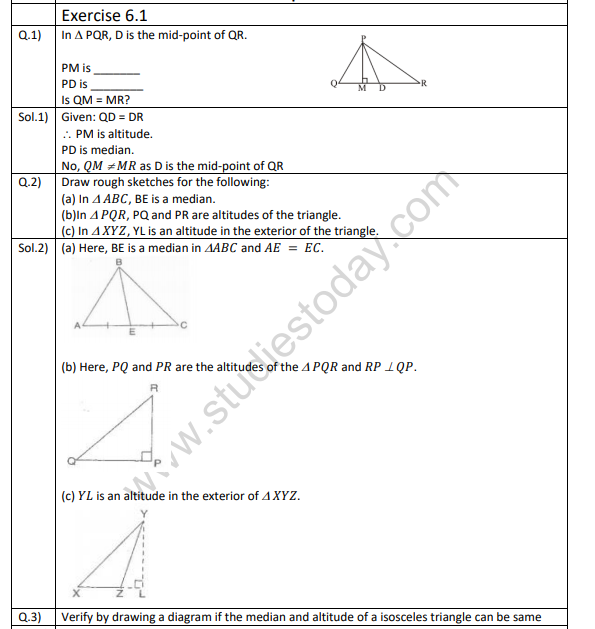Click for more Mathematics Study Material
 NCERT Solutions Class 7 Mathematics Integers
 NCERT Solutions Class 7 Mathematics Fractions and Decimals
 NCERT Solutions Class 7 Mathematics Data Handling
 NCERT Solutions Class 7 Mathematics Simple Equations
 NCERT Solutions Class 7 Mathematics Lines and angles
 NCERT Solutions Class 7 Mathematics Triangle and its properties
 NCERT Solutions Class 7 Mathematics Congruence of Triangle
 NCERT Solutions Class 7 Mathematics Comparing Quantities
 NCERT Solutions Class 7 Mathematics Rational Numbers
 NCERT Solutions Class 7 Mathematics Practical Geometry
 NCERT Solutions Class 7 Mathematics Perimeter and Area
 NCERT Solutions Class 7 Mathematics Algebraic Expressions
 NCERT Solutions Class 7 Mathematics Exponents and Power
 NCERT Solutions Class 7 Mathematics Symmetry
 NCERT Solutions Class 7 Mathematics Visualizing Solid Shapes

# NCERT Solutions Class 7 Mathematics Symmetry

NCERT Solutions Class 7 Mathematics Symmetry. The NCERT solutions have been made by teacher of one of the best CBSE school in India. These solutions have been made to give detailed answers and expanations of the concepts which can be easily understood by the students. Refer to other links also to download mathematics NCERT solutions, worksheets and test papers.Click for more Mathematics Study Material
 NCERT Solutions Class 7 Mathematics Integers
 NCERT Solutions Class 7 Mathematics Fractions and Decimals
 NCERT Solutions Class 7 Mathematics Data Handling
 NCERT Solutions Class 7 Mathematics Simple Equations
 NCERT Solutions Class 7 Mathematics Lines and angles
 NCERT Solutions Class 7 Mathematics Triangle and its properties
 NCERT Solutions Class 7 Mathematics Congruence of Triangle
 NCERT Solutions Class 7 Mathematics Comparing Quantities
 NCERT Solutions Class 7 Mathematics Rational Numbers
 NCERT Solutions Class 7 Mathematics Practical Geometry
 NCERT Solutions Class 7 Mathematics Perimeter and Area
 NCERT Solutions Class 7 Mathematics Algebraic Expressions
 NCERT Solutions Class 7 Mathematics Exponents and Power
 NCERT Solutions Class 7 Mathematics Symmetry
 NCERT Solutions Class 7 Mathematics Visualizing Solid Shapes

# NCERT Solutions Class 7 Mathematics Simple Equations

NCERT Solutions Class 7 Mathematics Simple Equations. The NCERT solutions have been made by teacher of one of the best CBSE school in India. These solutions have been made to give detailed answers and expanations of the concepts which can be easily understood by the students. Refer to other links also to download mathematics NCERT solutions, worksheets and test papers.Click for more Mathematics Study Material
 NCERT Solutions Class 7 Mathematics Integers
 NCERT Solutions Class 7 Mathematics Fractions and Decimals
 NCERT Solutions Class 7 Mathematics Data Handling
 NCERT Solutions Class 7 Mathematics Simple Equations
 NCERT Solutions Class 7 Mathematics Lines and angles
 NCERT Solutions Class 7 Mathematics Triangle and its properties
 NCERT Solutions Class 7 Mathematics Congruence of Triangle
 NCERT Solutions Class 7 Mathematics Comparing Quantities
 NCERT Solutions Class 7 Mathematics Rational Numbers
 NCERT Solutions Class 7 Mathematics Practical Geometry
 NCERT Solutions Class 7 Mathematics Perimeter and Area
 NCERT Solutions Class 7 Mathematics Algebraic Expressions
 NCERT Solutions Class 7 Mathematics Exponents and Power
 NCERT Solutions Class 7 Mathematics Symmetry
 NCERT Solutions Class 7 Mathematics Visualizing Solid Shapes

# NCERT Solutions Class 7 Mathematics Rational Numbers

NCERT Solutions Class 7 Mathematics Rational Numbers. The NCERT solutions have been made by teacher of one of the best CBSE school in India. These solutions have been made to give detailed answers and expanations of the concepts which can be easily understood by the students. Refer to other links also to download mathematics NCERT solutions, worksheets and test papers.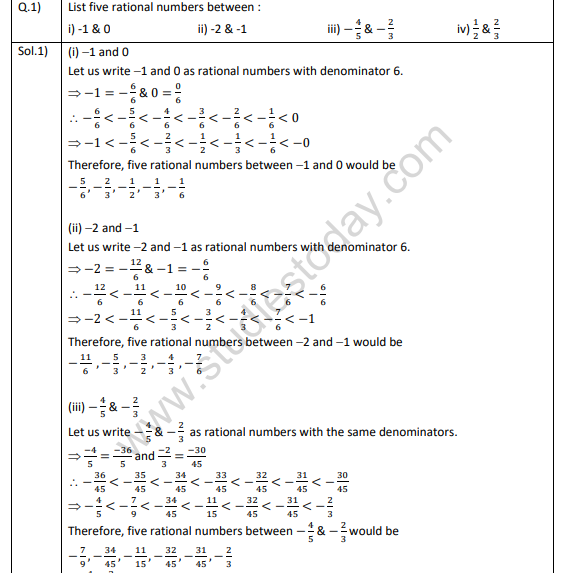Click for more Mathematics Study Material
 NCERT Solutions Class 7 Mathematics Integers
 NCERT Solutions Class 7 Mathematics Fractions and Decimals
 NCERT Solutions Class 7 Mathematics Data Handling
 NCERT Solutions Class 7 Mathematics Simple Equations
 NCERT Solutions Class 7 Mathematics Lines and angles
 NCERT Solutions Class 7 Mathematics Triangle and its properties
 NCERT Solutions Class 7 Mathematics Congruence of Triangle
 NCERT Solutions Class 7 Mathematics Comparing Quantities
 NCERT Solutions Class 7 Mathematics Rational Numbers
 NCERT Solutions Class 7 Mathematics Practical Geometry
 NCERT Solutions Class 7 Mathematics Perimeter and Area
 NCERT Solutions Class 7 Mathematics Algebraic Expressions
 NCERT Solutions Class 7 Mathematics Exponents and Power
 NCERT Solutions Class 7 Mathematics Symmetry
 NCERT Solutions Class 7 Mathematics Visualizing Solid Shapes

# NCERT Solutions Class 7 Mathematics Practical Geometry

NCERT Solutions Class 7 Mathematics Practical Geometry. The NCERT solutions have been made by teacher of one of the best CBSE school in India. These solutions have been made to give detailed answers and expanations of the concepts which can be easily understood by the students. Refer to other links also to download mathematics NCERT solutions, worksheets and test papers.Click for more Mathematics Study Material
 NCERT Solutions Class 7 Mathematics Integers
 NCERT Solutions Class 7 Mathematics Fractions and Decimals
 NCERT Solutions Class 7 Mathematics Data Handling
 NCERT Solutions Class 7 Mathematics Simple Equations
 NCERT Solutions Class 7 Mathematics Lines and angles
 NCERT Solutions Class 7 Mathematics Triangle and its properties
 NCERT Solutions Class 7 Mathematics Congruence of Triangle
 NCERT Solutions Class 7 Mathematics Comparing Quantities
 NCERT Solutions Class 7 Mathematics Rational Numbers
 NCERT Solutions Class 7 Mathematics Practical Geometry
 NCERT Solutions Class 7 Mathematics Perimeter and Area
 NCERT Solutions Class 7 Mathematics Algebraic Expressions
 NCERT Solutions Class 7 Mathematics Exponents and Power
 NCERT Solutions Class 7 Mathematics Symmetry
 NCERT Solutions Class 7 Mathematics Visualizing Solid Shapes

# NCERT Solutions Class 7 Mathematics Perimeter and Area

NCERT Solutions Class 7 Mathematics Perimeter and Area. The NCERT solutions have been made by teacher of one of the best CBSE school in India. These solutions have been made to give detailed answers and expanations of the concepts which can be easily understood by the students. Refer to other links also to download mathematics NCERT solutions, worksheets and test papers.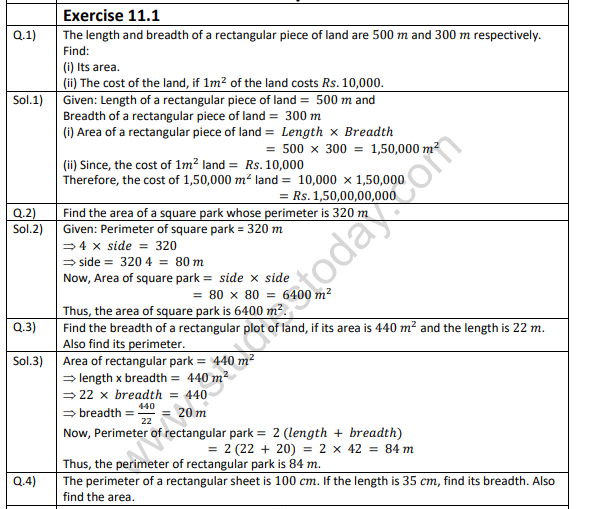Click for more Mathematics Study Material
 NCERT Solutions Class 7 Mathematics Integers
 NCERT Solutions Class 7 Mathematics Fractions and Decimals
 NCERT Solutions Class 7 Mathematics Data Handling
 NCERT Solutions Class 7 Mathematics Simple Equations
 NCERT Solutions Class 7 Mathematics Lines and angles
 NCERT Solutions Class 7 Mathematics Triangle and its properties
 NCERT Solutions Class 7 Mathematics Congruence of Triangle
 NCERT Solutions Class 7 Mathematics Comparing Quantities
 NCERT Solutions Class 7 Mathematics Rational Numbers
 NCERT Solutions Class 7 Mathematics Practical Geometry
 NCERT Solutions Class 7 Mathematics Perimeter and Area
 NCERT Solutions Class 7 Mathematics Algebraic Expressions
 NCERT Solutions Class 7 Mathematics Exponents and Power
 NCERT Solutions Class 7 Mathematics Symmetry
 NCERT Solutions Class 7 Mathematics Visualizing Solid Shapes

# NCERT Solutions Class 7 Mathematics Lines and angles

NCERT Solutions Class 7 Mathematics Lines and angles. The NCERT solutions have been made by teacher of one of the best CBSE school in India. These solutions have been made to give detailed answers and expanations of the concepts which can be easily understood by the students. Refer to other links also to download mathematics NCERT solutions, worksheets and test papers.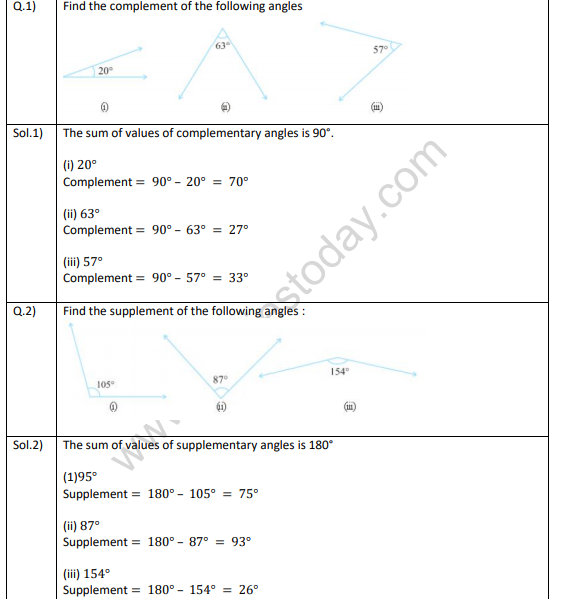Click for more Mathematics Study Material
 NCERT Solutions Class 7 Mathematics Integers
 NCERT Solutions Class 7 Mathematics Fractions and Decimals
 NCERT Solutions Class 7 Mathematics Data Handling
 NCERT Solutions Class 7 Mathematics Simple Equations
 NCERT Solutions Class 7 Mathematics Lines and angles
 NCERT Solutions Class 7 Mathematics Triangle and its properties
 NCERT Solutions Class 7 Mathematics Congruence of Triangle
 NCERT Solutions Class 7 Mathematics Comparing Quantities
 NCERT Solutions Class 7 Mathematics Rational Numbers
 NCERT Solutions Class 7 Mathematics Practical Geometry
 NCERT Solutions Class 7 Mathematics Perimeter and Area
 NCERT Solutions Class 7 Mathematics Algebraic Expressions
 NCERT Solutions Class 7 Mathematics Exponents and Power
 NCERT Solutions Class 7 Mathematics Symmetry
 NCERT Solutions Class 7 Mathematics Visualizing Solid Shapes

# NCERT Solutions Class 7 Mathematics Integers

NCERT Solutions Class 7 Mathematics Integers. The NCERT solutions have been made by teacher of one of the best CBSE school in India. These solutions have been made to give detailed answers and expanations of the concepts which can be easily understood by the students. Refer to other links also to download mathematics NCERT solutions, worksheets and test papers.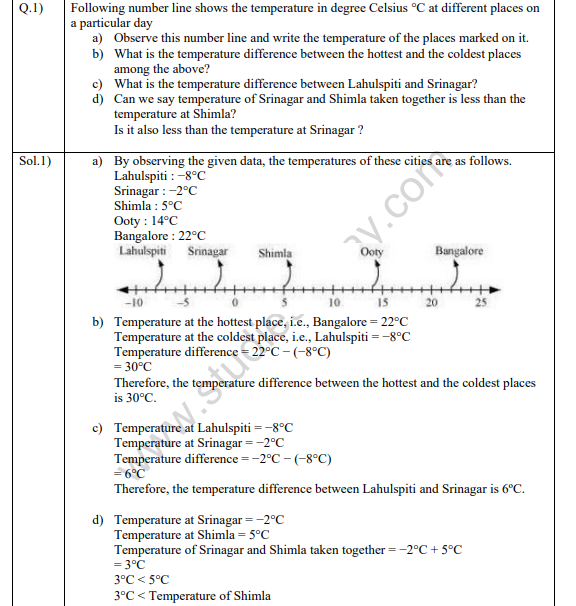Click for more Mathematics Study Material
 NCERT Solutions Class 7 Mathematics Integers
 NCERT Solutions Class 7 Mathematics Fractions and Decimals
 NCERT Solutions Class 7 Mathematics Data Handling
 NCERT Solutions Class 7 Mathematics Simple Equations
 NCERT Solutions Class 7 Mathematics Lines and angles
 NCERT Solutions Class 7 Mathematics Triangle and its properties
 NCERT Solutions Class 7 Mathematics Congruence of Triangle
 NCERT Solutions Class 7 Mathematics Comparing Quantities
 NCERT Solutions Class 7 Mathematics Rational Numbers
 NCERT Solutions Class 7 Mathematics Practical Geometry
 NCERT Solutions Class 7 Mathematics Perimeter and Area
 NCERT Solutions Class 7 Mathematics Algebraic Expressions
 NCERT Solutions Class 7 Mathematics Exponents and Power
 NCERT Solutions Class 7 Mathematics Symmetry
 NCERT Solutions Class 7 Mathematics Visualizing Solid Shapes

# NCERT Solutions Class 7 Mathematics Fractions and Decimals

NCERT Solutions Class 7 Mathematics Fractions and Decimals. The NCERT solutions have been made by teacher of one of the best CBSE school in India. These solutions have been made to give detailed answers and expanations of the concepts which can be easily understood by the students. Refer to other links also to download mathematics NCERT solutions, worksheets and test papers.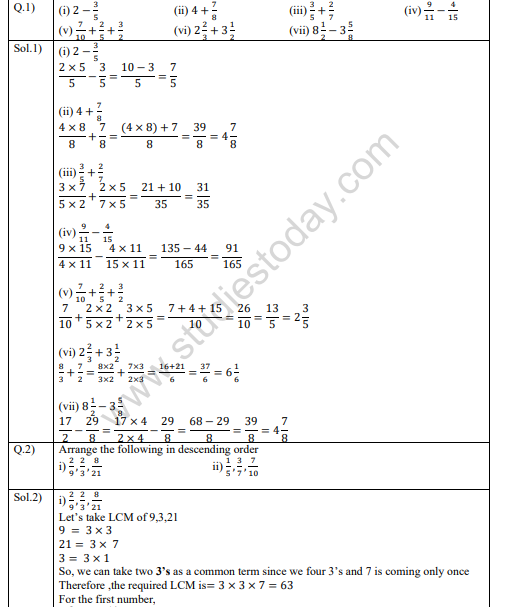Click for more Mathematics Study Material
 NCERT Solutions Class 7 Mathematics Integers
 NCERT Solutions Class 7 Mathematics Fractions and Decimals
 NCERT Solutions Class 7 Mathematics Data Handling
 NCERT Solutions Class 7 Mathematics Simple Equations
 NCERT Solutions Class 7 Mathematics Lines and angles
 NCERT Solutions Class 7 Mathematics Triangle and its properties
 NCERT Solutions Class 7 Mathematics Congruence of Triangle
 NCERT Solutions Class 7 Mathematics Comparing Quantities
 NCERT Solutions Class 7 Mathematics Rational Numbers
 NCERT Solutions Class 7 Mathematics Practical Geometry
 NCERT Solutions Class 7 Mathematics Perimeter and Area
 NCERT Solutions Class 7 Mathematics Algebraic Expressions
 NCERT Solutions Class 7 Mathematics Exponents and Power
 NCERT Solutions Class 7 Mathematics Symmetry
 NCERT Solutions Class 7 Mathematics Visualizing Solid Shapes

## Latest NCERT & CBSE News

Read the latest news and announcements from NCERT and CBSE below. Important updates relating to your studies which will help you to keep yourself updated with latest happenings in school level education. Keep yourself updated with all latest news and also read articles from teachers which will help you to improve your studies, increase motivation level and promote faster learning

### National Youth Day and Birth Anniversary of Swami Vivekananda

Ministry of Education, Govt. of India vide D.O No. 12-4/2021-IS.4 dated 04.01.2022 intimated that 12 January 2022 will be celebrated as “National Youth Day” and “Birth Anniversary of Swami Vivekananda”.   All Schools affiliated to CBSE may celebrate 12 January 2022 as...

### CBSE Science Challenge 2021 22

Science is inexplicably linked with our lives and helps us to understand the world around us better. Scientific and technological developments contribute to progress and help improve our standards of living. By engaging with this subject, students learn to think, solve...

### CBSE Term 2 Board Examinations

CBSE vide Circular No.Acad-51/2021 dated 5th July, 2021, notified that in the session 2021-2022, Board Examinations would be conducted in two terms, i.e.. Term I and Term II. This decision was taken due to the uncertainty arising out of COVID 19 Pandemic. Term I...

### Pariksha Pe Charcha 2022

The 5th edition of Pariskhas Pe Charcha the unique interactive program of Hon’ble Prime Minister with students teaches and parents will be held through virtual mode in February, 2022. In order to select participants who will be featured in Pariksha Pe Charcha programme...

### Celebration of Matribhasha Diwas Mother Language day

UNESCO has declared 21st February of every year to be celebrated as International Mother Language day to promote dissemination of Mother Language of all, create awareness of linguistic and cultural traditions and diversity across the world and to inspire solidarity...

### Surya Namaskar Project on 75th Anniversary of Independence Day

Ministry of Education, Govt of India vide letter No. F.No. 12-5/2020-IS-4 dated 16.12.2021 has intimated that under the banner Azadi ka Amrit Mahotsav the National Yogasanasports Federation has decided to run a project of 750 million Surya Namaskar from 01 January 2022...